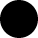﻿Add Custom Images To PDF Document | VB.NETAdd Custom Images To PDF Document | VB.NET ByteScout Barcode SDK

Add Custom Images To PDF Document | VB.NETProgram.vb:

VB
```Imports System.Diagnostics
Imports System.Drawing
Imports Bytescout.BarCode

' This example demostrates adding of barcode together with some custom images (e.g. target marks) to PDF document.
' Also shows the manual calculation of barcode size from inches to pixels and document units (points).
' Task: place Code39 barcode of 2" x 3/8" size at 2" from top-right corner and two target marks at 1" from top-right and bottom-left corners.

Class Program
Friend Shared Sub Main(args As String())

' Create Barcode instance and set it up
Dim barcode As New Barcode("demo", "demo")
barcode.Symbology = SymbologyType.Code39
barcode.Value = "00090112"
barcode.DrawCaption = True
barcode.CaptionFont = New Font("Courier", 12.0F, FontStyle.Bold, GraphicsUnit.Point)
barcode.DrawQuietZones = False
barcode.ResolutionX = 300 ' High resolution for better quality on document scaling and printing.
barcode.ResolutionY = 300

' Compute barcode image dimension from inches to pixels at 300 DPI:

Dim barHeight As Integer = CInt(Math.Truncate(3.0F / 8 * 300)) ' = 3/8" * 300 DPI = height of bars
Dim captionHeight As Integer = CInt(Math.Truncate(barcode.CaptionFont.GetHeight(barcode.ResolutionY)))
Dim captionGap As Integer = CInt(Math.Truncate(1.0F / 8 * 300)) ' = 1/8" gap

Dim barcodeImageWidth As Integer = 2 * 300 ' = 2" * 300 DPI = width of barcode
Dim barcodeImageHeight As Integer = barHeight + captionGap + captionHeight + 28 ' 28 = height of watermark text (painted in Trial version only)

' Get final barcode image:

barcode.BarHeight = barHeight
barcode.FitInto(barcodeImageWidth, barcodeImageHeight, UnitOfMeasure.Pixel)
Dim barcodeImage As Image = barcode.GetImage()

' Arrays of images and points to apply to PDF document
Dim images As Image() = New Image(2) {}
Dim points As Point() = New Point(2) {}

' Compute coordinates of barcode image and target marks ar 72 DPI
' (page size is 8.5" x 11", PDF document resolution is always 72 DPI):

Dim x As Integer = CInt(Math.Truncate(8.5F * 72 - 2 * 72 - barcodeImageWidth / 300.0F * 72)) ' = page width - 2" - barcodeImageWidth at 72 DPI = X coordinate to put the barcode image
Dim y As Integer = 2 * 72 ' = 2" - Y coordinate to put the barcode image

' Put barcode image into array
images(0) = barcodeImage
points(0) = New Point(x, y)

' TargetMark.png is 1/8" 300 DPI image (38x38 pixels)
Dim targetMarkImage As Image = Image.FromFile("TargetMark.png")

' Coordinates of top-right target mark
x = CInt(Math.Truncate(8.5F * 72 - 1 * 72 - 1.0F / 8 * 72)) ' = pageWidth - 1" - target mark width (1/8") at 72DPI = X coordinate to put the barcode image
y = 1 * 72 ' = 1" - Y coordinate to put the barcode image

' Put first target mark image into array
images(1) = targetMarkImage
points(1) = New Point(x, y)

' Coordinates of bottom-left target mark
x = 1 * 72 ' = 1"
y = CInt(Math.Truncate(11.0F * 72 - 1 * 72 - 1.0F / 8 * 72)) ' = page height - 1" - target mark height (1/8") at 72DPI

' Put second target mark image into array
images(2) = targetMarkImage
points(2) = New Point(x, y)

' Draw images on all PDF document pages.
barcode.DrawImagesToPDF("wikipedia.pdf", -1, images, points, "result.pdf")

' Cleanup
barcodeImage.Dispose()
targetMarkImage.Dispose()
barcode.Dispose()

' Open the result document in default associated application
Process.Start("result.pdf")

End Sub

End Class
End Namespace```